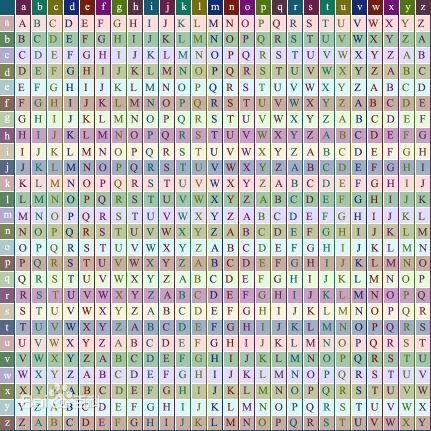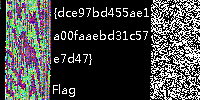2 篇文章 0 订阅

首届广西网络安全技术大赛初赛通关攻略

第一类：密码学

第二题 其实很简单（500分）

题目描述第二类：安全杂项

第二题 小明的保险箱（200分？）

题目附件（这里显示的图片可能会被处理过，导致丢失一些信息，想测试的最好是去云盘下载吧）

第四题 静静听这么好听的歌（388分）

附件里有一段代码：
fid=fopen('33.wav','rb');
n=length(a)-44;
fclose(fid);
[row col]=size(io);
wi=io(:);
if row*col>n
error('文件太小');
end
watermarkedaudio=a;
watermarklength=row*col;
for k=1:row*col
watermarkedaudio(44+k)=bitset(watermarkedaudio(44+k),1,wi(k));
end
figure;
subplot(2,1,1);plot(a);
subplot(2,1,2);plot(watermarkedaudio);
fid = fopen('2.wav', 'wb');
fwrite(fid,watermarkedaudio,'uchar');
fclose(fid);
解法第三类：WEB安全

第五题 贪食蛇（250分？）

贪食蛇代码中加密过的代码：
ﾟωﾟﾉ = /｀ｍ´）ﾉ ~┻━┻   //*´∇｀*/['_']; o = (ﾟｰﾟ) = _ = 3; c = (ﾟΘﾟ) = (ﾟｰﾟ) - (ﾟｰﾟ); (ﾟДﾟ) = (ﾟΘﾟ) = (o ^ _ ^ o) / (o ^ _ ^ o); (ﾟДﾟ) = { ﾟΘﾟ: '_', ﾟωﾟﾉ: ((ﾟωﾟﾉ == 3) + '_')[ﾟΘﾟ], ﾟｰﾟﾉ: (ﾟωﾟﾉ + '_')[o ^ _ ^ o - (ﾟΘﾟ)], ﾟДﾟﾉ: ((ﾟｰﾟ == 3) + '_')[ﾟｰﾟ] }; (ﾟДﾟ)[ﾟΘﾟ] = ((ﾟωﾟﾉ == 3) + '_')[c ^ _ ^ o]; (ﾟДﾟ)['c'] = ((ﾟДﾟ) + '_')[(ﾟｰﾟ) + (ﾟｰﾟ) - (ﾟΘﾟ)]; (ﾟДﾟ)['o'] = ((ﾟДﾟ) + '_')[ﾟΘﾟ]; (ﾟoﾟ) = (ﾟДﾟ)['c'] + (ﾟДﾟ)['o'] + (ﾟωﾟﾉ + '_')[ﾟΘﾟ] + ((ﾟωﾟﾉ == 3) + '_')[ﾟｰﾟ] + ((ﾟДﾟ) + '_')[(ﾟｰﾟ) + (ﾟｰﾟ)] + ((ﾟｰﾟ == 3) + '_')[ﾟΘﾟ] + ((ﾟｰﾟ == 3) + '_')[(ﾟｰﾟ) - (ﾟΘﾟ)] + (ﾟДﾟ)['c'] + ((ﾟДﾟ) + '_')[(ﾟｰﾟ) + (ﾟｰﾟ)] + (ﾟДﾟ)['o'] + ((ﾟｰﾟ == 3) + '_')[ﾟΘﾟ]; (ﾟДﾟ)['_'] = (o ^ _ ^ o)[ﾟoﾟ][ﾟoﾟ]; (ﾟεﾟ) = ((ﾟｰﾟ == 3) + '_')[ﾟΘﾟ] + (ﾟДﾟ).ﾟДﾟﾉ + ((ﾟДﾟ) + '_')[(ﾟｰﾟ) + (ﾟｰﾟ)] + ((ﾟｰﾟ == 3) + '_')[o ^ _ ^ o - ﾟΘﾟ] + ((ﾟｰﾟ == 3) + '_')[ﾟΘﾟ] + (ﾟωﾟﾉ + '_')[ﾟΘﾟ]; (ﾟｰﾟ) += (ﾟΘﾟ); (ﾟДﾟ)[ﾟεﾟ] = '\\'; (ﾟДﾟ).ﾟΘﾟﾉ = (ﾟДﾟ + ﾟｰﾟ)[o ^ _ ^ o - (ﾟΘﾟ)]; (oﾟｰﾟo) = (ﾟωﾟﾉ + '_')[c ^ _ ^ o]; (ﾟДﾟ)[ﾟoﾟ] = '\"'; (ﾟДﾟ)['_']((ﾟДﾟ)['_'](ﾟεﾟ + (ﾟДﾟ)[ﾟoﾟ] + (ﾟДﾟ)[ﾟεﾟ] + (ﾟΘﾟ) + ((o ^ _ ^ o) + (o ^ _ ^ o)) + ((ﾟｰﾟ) + (o ^ _ ^ o)) + (ﾟДﾟ)[ﾟεﾟ] + (ﾟΘﾟ) + ((ﾟｰﾟ) + (ﾟΘﾟ)) + (ﾟΘﾟ) + (ﾟДﾟ)[ﾟεﾟ] + (ﾟΘﾟ) + ((ﾟｰﾟ) + (ﾟΘﾟ)) + ((o ^ _ ^ o) + (o ^ _ ^ o)) + (ﾟДﾟ)[ﾟεﾟ] + (ﾟΘﾟ) + (ﾟｰﾟ) + (ﾟｰﾟ) + (ﾟДﾟ)[ﾟεﾟ] + (ﾟΘﾟ) + ((ﾟｰﾟ) + (ﾟΘﾟ)) + ((ﾟｰﾟ) + (o ^ _ ^ o)) + (ﾟДﾟ)[ﾟεﾟ] + (ﾟΘﾟ) + ((o ^ _ ^ o) + (o ^ _ ^ o)) + ((ﾟｰﾟ) + (o ^ _ ^ o)) + (ﾟДﾟ)[ﾟεﾟ] + (ﾟΘﾟ) + (o ^ _ ^ o) + (o ^ _ ^ o) + (ﾟДﾟ)[ﾟεﾟ] + (ﾟｰﾟ) + ((ﾟｰﾟ) + (o ^ _ ^ o)) + (ﾟДﾟ)[ﾟεﾟ] + (ﾟΘﾟ) + (ﾟｰﾟ) + ((o ^ _ ^ o) + (o ^ _ ^ o)) + (ﾟДﾟ)[ﾟεﾟ] + (ﾟΘﾟ) + ((ﾟｰﾟ) + (ﾟΘﾟ)) + (ﾟｰﾟ) + (ﾟДﾟ)[ﾟεﾟ] + (ﾟΘﾟ) + (ﾟｰﾟ) + (ﾟΘﾟ) + (ﾟДﾟ)[ﾟεﾟ] + (ﾟΘﾟ) + (ﾟｰﾟ) + ((ﾟｰﾟ) + (o ^ _ ^ o)) + (ﾟДﾟ)[ﾟεﾟ] + (ﾟｰﾟ) + ((ﾟｰﾟ) + (o ^ _ ^ o)) + (ﾟДﾟ)[ﾟεﾟ] + (ﾟΘﾟ) + (o ^ _ ^ o) + ((ﾟｰﾟ) + (ﾟΘﾟ)) + (ﾟДﾟ)[ﾟεﾟ] + (ﾟｰﾟ) + (c ^ _ ^ o) + (ﾟДﾟ)[ﾟεﾟ] + ((ﾟｰﾟ) + (o ^ _ ^ o)) + ((ﾟｰﾟ) + (ﾟΘﾟ)) + (ﾟДﾟ)[ﾟεﾟ] + (ﾟｰﾟ) + (c ^ _ ^ o) + (ﾟДﾟ)[ﾟεﾟ] + (ﾟｰﾟ) + ((ﾟｰﾟ) + (o ^ _ ^ o)) + (ﾟДﾟ)[ﾟεﾟ] + (ﾟΘﾟ) + (c ^ _ ^ o) + ((o ^ _ ^ o) + (o ^ _ ^ o)) + (ﾟДﾟ)[ﾟεﾟ] + (ﾟΘﾟ) + ((ﾟｰﾟ) + (ﾟΘﾟ)) + (ﾟｰﾟ) + (ﾟДﾟ)[ﾟεﾟ] + (ﾟΘﾟ) + (ﾟｰﾟ) + (ﾟΘﾟ) + (ﾟДﾟ)[ﾟεﾟ] + (ﾟΘﾟ) + (ﾟｰﾟ) + ((ﾟｰﾟ) + (o ^ _ ^ o)) + (ﾟДﾟ)[ﾟεﾟ] + (ﾟΘﾟ) + ((ﾟｰﾟ) + (o ^ _ ^ o)) + (o ^ _ ^ o) + (ﾟДﾟ)[ﾟεﾟ] + ((o ^ _ ^ o) + (o ^ _ ^ o)) + ((o ^ _ ^ o) + (o ^ _ ^ o)) + (ﾟДﾟ)[ﾟεﾟ] + (ﾟΘﾟ) + (ﾟｰﾟ) + ((o ^ _ ^ o) - (ﾟΘﾟ)) + (ﾟДﾟ)[ﾟεﾟ] + ((o ^ _ ^ o) + (o ^ _ ^ o)) + (ﾟｰﾟ) + (ﾟДﾟ)[ﾟεﾟ] + ((ﾟｰﾟ) + (o ^ _ ^ o)) + (c ^ _ ^ o) + (ﾟДﾟ)[ﾟεﾟ] + ((o ^ _ ^ o) + (o ^ _ ^ o)) + (c ^ _ ^ o) + (ﾟДﾟ)[ﾟεﾟ] + ((o ^ _ ^ o) + (o ^ _ ^ o)) + ((ﾟｰﾟ) + (o ^ _ ^ o)) + (ﾟДﾟ)[ﾟεﾟ] + ((o ^ _ ^ o) + (o ^ _ ^ o)) + ((o ^ _ ^ o) - (ﾟΘﾟ)) + (ﾟДﾟ)[ﾟεﾟ] + ((o ^ _ ^ o) + (o ^ _ ^ o)) + ((ﾟｰﾟ) + (o ^ _ ^ o)) + (ﾟДﾟ)[ﾟεﾟ] + ((o ^ _ ^ o) + (o ^ _ ^ o)) + (o ^ _ ^ o) + (ﾟДﾟ)[ﾟεﾟ] + (ﾟΘﾟ) + (ﾟｰﾟ) + (ﾟΘﾟ) + (ﾟДﾟ)[ﾟεﾟ] + (ﾟΘﾟ) + (ﾟｰﾟ) + ((o ^ _ ^ o) + (o ^ _ ^ o)) + (ﾟДﾟ)[ﾟεﾟ] + (ﾟΘﾟ) + (ﾟｰﾟ) + (ﾟｰﾟ) + (ﾟДﾟ)[ﾟεﾟ] + (ﾟΘﾟ) + (ﾟｰﾟ) + ((o ^ _ ^ o) + (o ^ _ ^ o)) + (ﾟДﾟ)[ﾟεﾟ] + (ﾟΘﾟ) + (ﾟｰﾟ) + ((o ^ _ ^ o) + (o ^ _ ^ o)) + (ﾟДﾟ)[ﾟεﾟ] + (ﾟΘﾟ) + (ﾟｰﾟ) + (o ^ _ ^ o) + (ﾟДﾟ)[ﾟεﾟ] + ((o ^ _ ^ o) + (o ^ _ ^ o)) + (ﾟｰﾟ) + (ﾟДﾟ)[ﾟεﾟ] + ((o ^ _ ^ o) + (o ^ _ ^ o)) + (ﾟｰﾟ) + (ﾟДﾟ)[ﾟεﾟ] + ((o ^ _ ^ o) + (o ^ _ ^ o)) + ((o ^ _ ^ o) - (ﾟΘﾟ)) + (ﾟДﾟ)[ﾟεﾟ] + ((o ^ _ ^ o) + (o ^ _ ^ o)) + ((o ^ _ ^ o) + (o ^ _ ^ o)) + (ﾟДﾟ)[ﾟεﾟ] + (ﾟΘﾟ) + (ﾟｰﾟ) + ((o ^ _ ^ o) - (ﾟΘﾟ)) + (ﾟДﾟ)[ﾟεﾟ] + ((o ^ _ ^ o) + (o ^ _ ^ o)) + ((ﾟｰﾟ) + (o ^ _ ^ o)) + (ﾟДﾟ)[ﾟεﾟ] + ((ﾟｰﾟ) + (o ^ _ ^ o)) + (ﾟΘﾟ) + (ﾟДﾟ)[ﾟεﾟ] + ((o ^ _ ^ o) + (o ^ _ ^ o)) + (c ^ _ ^ o) + (ﾟДﾟ)[ﾟεﾟ] + (ﾟΘﾟ) + (ﾟｰﾟ) + (ﾟｰﾟ) + (ﾟДﾟ)[ﾟεﾟ] + (ﾟΘﾟ) + (ﾟｰﾟ) + ((ﾟｰﾟ) + (ﾟΘﾟ)) + (ﾟДﾟ)[ﾟεﾟ] + (ﾟΘﾟ) + (ﾟｰﾟ) + ((o ^ _ ^ o) - (ﾟΘﾟ)) + (ﾟДﾟ)[ﾟεﾟ] + (ﾟΘﾟ) + (ﾟｰﾟ) + (o ^ _ ^ o) + (ﾟДﾟ)[ﾟεﾟ] + (ﾟΘﾟ) + (ﾟｰﾟ) + (ﾟｰﾟ) + (ﾟДﾟ)[ﾟεﾟ] + ((o ^ _ ^ o) + (o ^ _ ^ o)) + ((o ^ _ ^ o) - (ﾟΘﾟ)) + (ﾟДﾟ)[ﾟεﾟ] + (ﾟΘﾟ) + (ﾟｰﾟ) + ((o ^ _ ^ o) - (ﾟΘﾟ)) + (ﾟДﾟ)[ﾟεﾟ] + ((ﾟｰﾟ) + (o ^ _ ^ o)) + (ﾟΘﾟ) + (ﾟДﾟ)[ﾟεﾟ] + ((o ^ _ ^ o) + (o ^ _ ^ o)) + ((o ^ _ ^ o) + (o ^ _ ^ o)) + (ﾟДﾟ)[ﾟεﾟ] + (ﾟΘﾟ) + ((ﾟｰﾟ) + (o ^ _ ^ o)) + ((ﾟｰﾟ) + (ﾟΘﾟ)) + (ﾟДﾟ)[ﾟεﾟ] + (ﾟｰﾟ) + ((ﾟｰﾟ) + (o ^ _ ^ o)) + (ﾟДﾟ)[ﾟεﾟ] + ((ﾟｰﾟ) + (o ^ _ ^ o)) + (o ^ _ ^ o) + (ﾟДﾟ)[ﾟεﾟ] + (ﾟΘﾟ) + ((o ^ _ ^ o) - (ﾟΘﾟ)) + (ﾟДﾟ)[ﾟεﾟ] + (ﾟΘﾟ) + (ﾟｰﾟ) + (o ^ _ ^ o) + (ﾟДﾟ)[ﾟεﾟ] + (ﾟΘﾟ) + ((ﾟｰﾟ) + (ﾟΘﾟ)) + ((ﾟｰﾟ) + (o ^ _ ^ o)) + (ﾟДﾟ)[ﾟεﾟ] + (ﾟΘﾟ) + ((ﾟｰﾟ) + (ﾟΘﾟ)) + ((o ^ _ ^ o) + (o ^ _ ^ o)) + (ﾟДﾟ)[ﾟεﾟ] + (ﾟΘﾟ) + ((o ^ _ ^ o) + (o ^ _ ^ o)) + (o ^ _ ^ o) + (ﾟДﾟ)[ﾟεﾟ] + (ﾟΘﾟ) + ((ﾟｰﾟ) + (ﾟΘﾟ)) + ((ﾟｰﾟ) + (o ^ _ ^ o)) + (ﾟДﾟ)[ﾟεﾟ] + (ﾟΘﾟ) + ((ﾟｰﾟ) + (ﾟΘﾟ)) + (ﾟｰﾟ) + (ﾟДﾟ)[ﾟεﾟ] + (ﾟΘﾟ) + (ﾟｰﾟ) + ((ﾟｰﾟ) + (ﾟΘﾟ)) + (ﾟДﾟ)[ﾟεﾟ] + ((ﾟｰﾟ) + (ﾟΘﾟ)) + ((o ^ _ ^ o) + (o ^ _ ^ o)) + (ﾟДﾟ)[ﾟεﾟ] + (ﾟΘﾟ) + ((ﾟｰﾟ) + (ﾟΘﾟ)) + (ﾟｰﾟ) + (ﾟДﾟ)[ﾟεﾟ] + (ﾟΘﾟ) + ((ﾟｰﾟ) + (ﾟΘﾟ)) + ((ﾟｰﾟ) + (o ^ _ ^ o)) + (ﾟДﾟ)[ﾟεﾟ] + (ﾟΘﾟ) + (ﾟｰﾟ) + ((ﾟｰﾟ) + (o ^ _ ^ o)) + (ﾟДﾟ)[ﾟεﾟ] + ((ﾟｰﾟ) + (ﾟΘﾟ)) + (c ^ _ ^ o) + (ﾟДﾟ)[ﾟεﾟ] + (ﾟｰﾟ) + ((o ^ _ ^ o) - (ﾟΘﾟ)) + (ﾟДﾟ)[ﾟεﾟ] + (ﾟΘﾟ) + (c ^ _ ^ o) + ((o ^ _ ^ o) + (o ^ _ ^ o)) + (ﾟДﾟ)[ﾟεﾟ] + (ﾟΘﾟ) + ((ﾟｰﾟ) + (ﾟΘﾟ)) + (ﾟｰﾟ) + (ﾟДﾟ)[ﾟεﾟ] + (ﾟΘﾟ) + (ﾟｰﾟ) + (ﾟΘﾟ) + (ﾟДﾟ)[ﾟεﾟ] + (ﾟΘﾟ) + (ﾟｰﾟ) + ((ﾟｰﾟ) + (o ^ _ ^ o)) + (ﾟДﾟ)[ﾟεﾟ] + (ﾟΘﾟ) + ((ﾟｰﾟ) + (o ^ _ ^ o)) + (o ^ _ ^ o) + (ﾟДﾟ)[ﾟεﾟ] + (ﾟｰﾟ) + (c ^ _ ^ o) + (ﾟДﾟ)[ﾟεﾟ] + (ﾟΘﾟ) + ((ﾟｰﾟ) + (ﾟΘﾟ)) + (c ^ _ ^ o) + (ﾟДﾟ)[ﾟεﾟ] + (ﾟΘﾟ) + (ﾟｰﾟ) + (ﾟΘﾟ) + (ﾟДﾟ)[ﾟεﾟ] + (ﾟΘﾟ) + ((ﾟｰﾟ) + (ﾟΘﾟ)) + (c ^ _ ^ o) + (ﾟДﾟ)[ﾟεﾟ] + (ﾟΘﾟ) + (ﾟｰﾟ) + (ﾟΘﾟ) + (ﾟДﾟ)[ﾟεﾟ] + (ﾟΘﾟ) + ((ﾟｰﾟ) + (ﾟΘﾟ)) + (c ^ _ ^ o) + (ﾟДﾟ)[ﾟεﾟ] + (ﾟΘﾟ) + (ﾟｰﾟ) + (ﾟΘﾟ) + (ﾟДﾟ)[ﾟεﾟ] + (ﾟΘﾟ) + ((ﾟｰﾟ) + (ﾟΘﾟ)) + (c ^ _ ^ o) + (ﾟДﾟ)[ﾟεﾟ] + (ﾟｰﾟ) + (c ^ _ ^ o) + (ﾟДﾟ)[ﾟεﾟ] + (ﾟΘﾟ) + ((o ^ _ ^ o) + (o ^ _ ^ o)) + ((ﾟｰﾟ) + (o ^ _ ^ o)) + (ﾟДﾟ)[ﾟεﾟ] + (ﾟΘﾟ) + ((o ^ _ ^ o) + (o ^ _ ^ o)) + ((o ^ _ ^ o) - (ﾟΘﾟ)) + (ﾟДﾟ)[ﾟεﾟ] + (ﾟΘﾟ) + ((ﾟｰﾟ) + (ﾟΘﾟ)) + ((ﾟｰﾟ) + (o ^ _ ^ o)) + (ﾟДﾟ)[ﾟεﾟ] + (ﾟΘﾟ) + ((ﾟｰﾟ) + (ﾟΘﾟ)) + ((o ^ _ ^ o) + (o ^ _ ^ o)) + (ﾟДﾟ)[ﾟεﾟ] + (ﾟΘﾟ) + (ﾟｰﾟ) + ((ﾟｰﾟ) + (o ^ _ ^ o)) + (ﾟДﾟ)[ﾟεﾟ] + (ﾟｰﾟ) + (ﾟΘﾟ) + (ﾟДﾟ)[ﾟεﾟ] + (ﾟｰﾟ) + (ﾟΘﾟ) + (ﾟДﾟ)[ﾟεﾟ] + (ﾟｰﾟ) + (c ^ _ ^ o) + (ﾟДﾟ)[ﾟεﾟ] + ((ﾟｰﾟ) + (o ^ _ ^ o)) + ((o ^ _ ^ o) - (ﾟΘﾟ)) + (ﾟДﾟ)[ﾟεﾟ] + ((ﾟｰﾟ) + (ﾟΘﾟ)) + (c ^ _ ^ o) + (ﾟДﾟ)[ﾟεﾟ] + (ﾟΘﾟ) + ((ﾟｰﾟ) + (o ^ _ ^ o)) + ((ﾟｰﾟ) + (ﾟΘﾟ)) + (ﾟДﾟ)[ﾟεﾟ] + (ﾟｰﾟ) + ((o ^ _ ^ o) - (ﾟΘﾟ)) + (ﾟДﾟ)[ﾟεﾟ] + ((ﾟｰﾟ) + (ﾟΘﾟ)) + (ﾟΘﾟ) + (ﾟДﾟ)[ﾟεﾟ] + ((ﾟｰﾟ) + (o ^ _ ^ o)) + (o ^ _ ^ o) + (ﾟДﾟ)[ﾟoﾟ])(ﾟΘﾟ))('_');
解法

flag就藏在前台js中。先分析贪吃蛇的代码，发现成功时会执行一句奇特的加密代码，我们运行它，得到：

Flag{ hahahah wrong!! :(}

"return"\167\151\156\144\157\167\133\47\146\154\141\147\47\135\40\75\40\47\106\154\141\147\173\66\142\64\70\60\67\62\67\63\141\146\144\146\146\143\64\64\62\66\142\67\71\60\144\145\142\143\144\62\142\71\66\175\47\73\12\143\157\156\163\157\154\145\56\154\157\147\50\42\106\154\141\147\173\40\150\141\150\141\150\141\150\40\167\162\157\156\147\41\41\40\72\50\175\42\51\73""

"window['flag'] = 'Flag{6b4807273afdffc4426b790debcd2b96}';
console.log("Flag{ hahahah wrong!! :(}");"

第十题 刀塔（300分？）

解法

flag位于http://靶机/flag.php，直接运行不会显示flag。
news页有目录穿越漏洞，它的地址大概是这样的：http://靶机/index.php?action=news&nid=1

http://靶机/index.php?action=news&nid=../*

溢出类

第一题 高水平溢出（500分）

点评

void __noreturn process()
{
while(true)
{
//这里是代码第一行，第一行，真的第一行！
scanf("%s", buff);
fflush(stdin);
printf(...); // 打印欢迎语
...
}
}

第四题 宝葫芦身（300分）

解法

unsigned char str[] = "dbapp";
*(unsigned char *)str = 0xFF;
*(unsigned char *)(str+1) = 0xE4;

逆向破解

39D09FFA4CFCC4CC
4EB5F3992391A1AE

第五题 helloword（100分）

解法

16进制编辑器打开classes.dex，抓取正则：/flag{\w+}/，得到flag：
flag{7631a988259a00816deda84afb29430a}

06-02
02-031万+

04-261725
02-031万+
08-18531
05-241389
08-19583
12-15
04-08
11-135万+
08-18268
04-191926
10-058838
09-121万+
04-167万+
01-212万+Happy0403

¥2 ¥4 ¥6 ¥10 ¥20余额支付 (余额：-- )扫码支付获取中扫码支付点击重新获取扫码支付1.余额是钱包充值的虚拟货币，按照1:1的比例进行支付金额的抵扣。
2.余额无法直接购买下载，可以购买VIP、C币套餐、付费专栏及课程。余额充值